# Suspension

(diff) ← Older revision | Latest revision (diff) | Newer revision → (diff)

of a topological space (CW-complex)The space (CW-complex)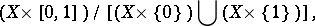whereis the unit interval and the slant line denotes the operation of identifying a subspace with one point. The suspension of a pointed space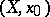is defined to be the pointed spaceThis is also known as a reduced or contracted suspension. A suspension is denoted by(or sometimes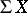). The correspondencedefines a functor from the category of topological (pointed) spaces into itself.

Since the suspension operation is a functor, one can define a homomorphism, which is also called the suspension. This homomorphism is identical with the composite of the homomorphism induced by the imbedding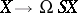and the Hurewicz isomorphism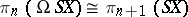, whereis the operation of forming loop spaces (cf. Loop space). For any homology theory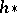(cohomology theory) one has an isomorphism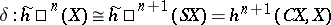that coincides with the connecting homomorphism of the exact sequence of the pair, where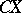is the cone over. The image of a class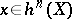under this isomorphism is known as the suspension ofand is denoted by(or).

The suspension of a cohomology operationis defined to be the cohomology operation whose action on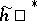coincides with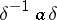, and whose action on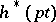coincides with that of.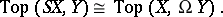The bijection above associates tothe mapping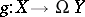which associates the loop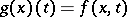to. This adjointness is compatible with the homology and thus also defines an adjunction for the category of pointed topological spaces and homotopy classes of mappings.